# Pythagorean Identities

The Pythagorean Identities are considered to be fundamental identities in trigonometry. They express the Pythagorean Theorem in trigonometric terms. The main Pythagorean Identity is: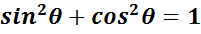Let's look at how it works. Given the unit circle, which has a radius of 1, and any point on the circle that creates the vertex of a right triangle can be represented by the coordinates (x, y)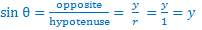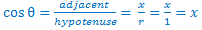Recall the Pythagorean Theorem is x2 + y2 = c2. Since the legs of the right triangle can be represented by sin θ and cos θ and the radius is the hypotenuse we can use the Pythagorean Theorem to derive sin2 θ + cos2 θ = 1.

From this fundamental identity we can derive equivalent identities such as:

• sin2 θ + cos2 θ = 1

• -cos2 θ     -cos2 θ    using the subtraction property of equality

 sin2 θ = 1 - cos2 θ

• sin2 θ + cos2 θ = 1

• -sin2 θ     -sin2 θ    using the subtraction property of equality

 cos2 θ = 1 - sin2 θ

•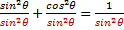using the division property of equality

• 1 + cot2 θ = csc2 θ    use substitution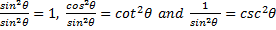-1-1use subtraction property of equality

 cot2 θ = csc2 θ - 1

Deriving the Pythagorean identities leads to understanding the basic trigonometric proofs. Recall that when solving an equation both sides of the equation may be manipulated to find a solution. However, when proving an identity only one side of the identity may be manipulated.

 Related Links: Math Trigonometry Cofunction Identities Sum and Difference of Angles Identities

To link to this Pythagorean Identities page, copy the following code to your site: